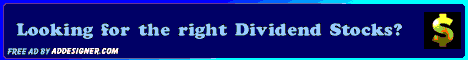Dividends4Life: Updated D4L-PreScreen.xls Model

## Updated D4L-PreScreen.xls Model

Posted by D4L | Saturday, December 27, 2008 | , | 0 comments »By far, my most used and most downloaded model is the D4L-PreScreen.xls model. I have just completed an update on it. This update includes the normal year-end changes, plus some rework on the Projected Information section. The changes are described below:

• Changed year from 2008 to 2009
• Updated the default MMA Yield to 3.54%
The 3.54% rate is an average of the last two years highest ending MMA rate that I held. The rate will fluctuate +/- 0.5% as MMA rates change. In addition, I will continue to compare the rate to The 20-Year Treasury Yield. On December 1st, this rate was 3.51%; however, it has continued to drop since then. I will monitor the rate and adjust accordingly in the future.

Projected Information Section
This section was significantly reworked and expanded. Recently, I noticed a logic flaw in the NPV of MMA Differential calculation. The NPV was being calculated on a series of differences between the cumulative values of the stock and MMA. I added an annual calculation (row 23), below the errant calculation (row 22), which I left and continue to calculate a NPV of. The errant calculation generally followed the correct annual calculation and when calculating a NPV allowed for a wider range. The new correct NPV is found in cell B50. In an effort to calibrate the correct calculation, I will watch both during 2009.

Previous models only included the No Price Appreciation calculation. In his version, I added two other alternatives: Price Appreciation = Dividend Growth and Dividends/Interest Not Reinvested. Each are described below:

No Price Appreciation
This model assumes that the share price remains constant, thus the dividend yield grows each year based on the dividend growth rate. It also assumes all dividends and interest are reinvested.

Price Appreciation = Dividend Growth
This model assumes that the share price grows at the dividend growth rate, thus the dividend yield remains constant. It also assumes all dividends and interest are reinvested.

Dividends/Interest Not Reinvested
This model is identical to the No Price Appreciation model, except the dividends and interest are not reinvested.

The NPV of MMA Differential and the Sum of MMA Differential for each of the above calculations is found on rows 50 and 51.

Of the various models, I think that the Price Appreciation = Dividend Growth model provides the most realist results, but I generally do not use it because it includes capital growth, which is not accessible unless you sell the security. This runs counter to a income-based buy-and-hold philosophy.

If you run across any bugs or glitches, please be sure to let me know.

Disclaimer
This model is for illustrative and educational purposes only. The author and Dividends4Life makes no claims or assertions as to the model's accuracy, completeness, appropriateness of use, or any other claim or assertion. You should not rely on this model or base any financial decisions on it.

Related Articles:

________________________________________________________________